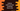# Python program to select an item randomly from a list## How to select an item randomly from a list in Python :

In python, we have a couple of different ways to select an item randomly from a list. In this post, I will show you different ways to do that with examples.

## Method 1 : By using random.choice() :

This is the easiest and recommended way to pick a random item from a list. choice() method is defined in the random module that we can use just right out of the box. This function takes one sequence as the argument and returns one random element from that sequence. If we pass one empty list, it will throw one IndexError. This method is defined as below :

``random.choice(seq)``

It returns one random value from the given sequence seq.

Let me show you one simple example of random.choice :

### Example of random.choice() :

``````import random
given_list = [1, 2, 3, 4, 5, 6, 7, 8, 9, 10]

print(random.choice(given_list))``````

If you run this program, it will print one random value from givenlist_ returned by random.choice. Each time, we will get one random value.

### Method 2: More secure way to get a random value by using secrets :

Python 3.6 introduced one new module called secrets with different methods to generate cryptographically strong numbers. secrets should be used instead of random if you need security to your program.

The method is choice i.e. secrets.choice that takes one sequence and returns one random element from a non empty sequence.

``secrets.choice(seq)``

If we rewrite the above example, it will give us similar outputs :

``````import secrets
given_list = [1, 2, 3, 4, 5, 6, 7, 8, 9, 10]

print(secrets.choice(given_list))``````

## Example : Get random value from a list of strings :

In a similar way, we can get a random value from a list of strings like below :

``````import secrets
import random

given_list = ['one', 'two', 'three', 'four', 'five', 'six']

print(secrets.choice(given_list))
print(random.choice(given_list))``````